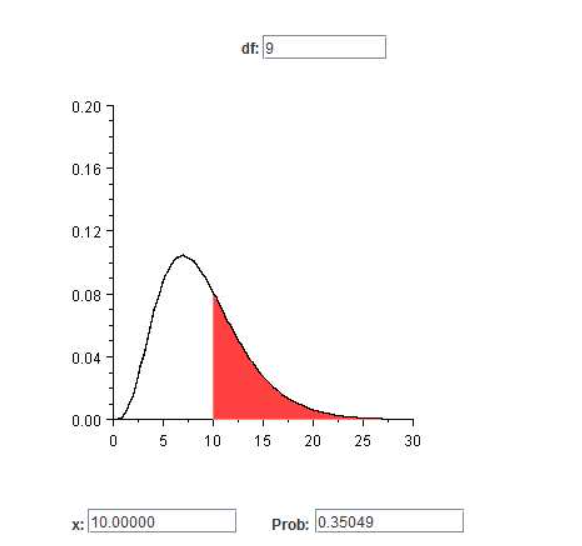### Solution Found!# Applet Exercisea Use the applet Chi-Square Probabilities

Chapter 7, Problem 23E

(choose chapter or problem)

Get Unlimited Answers! Check out our subscriptions
QUESTION:

Applet Exercise

Use the applet Chi-Square Probabilities and Quantiles to find $$P[Y>E(Y)]$$ when Y has $$\mathrm{X}^{2}$$ distributions with 10, 40, and 80 df.What did you notice about $$P[Y>E(Y)]$$ as the number of degrees of freedom increases as in part (a)?How does what you observed in part (b) relate to the shapes of the $$\mathrm{X}^{2}$$ densities that you obtained in Exercise 7.22?

Equation Transcription:Text Transcription:

P[Y>E(Y)]

X^2

P[Y>E(Y)]

X^2

QUESTION:

Applet Exercise

Use the applet Chi-Square Probabilities and Quantiles to find $$P[Y>E(Y)]$$ when Y has $$\mathrm{X}^{2}$$ distributions with 10, 40, and 80 df.What did you notice about $$P[Y>E(Y)]$$ as the number of degrees of freedom increases as in part (a)?How does what you observed in part (b) relate to the shapes of the $$\mathrm{X}^{2}$$ densities that you obtained in Exercise 7.22?

Equation Transcription:Text Transcription:

P[Y>E(Y)]

X^2

P[Y>E(Y)]

X^2

Step 1 of 5

a)

Lethas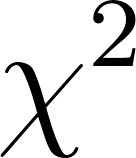distribution withdegrees of freedom.

Then,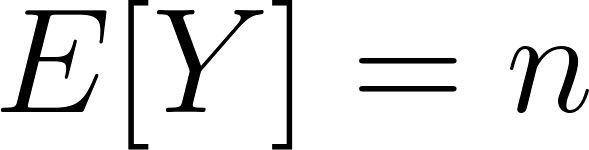and the degrees of freedom,Consider, then,and the degrees of freedom,Then,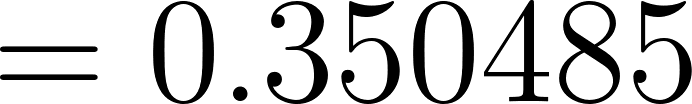(Using EXCEL command = chidist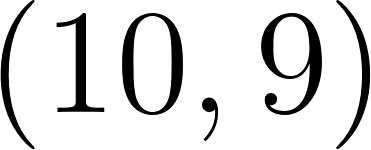)

The applet of the above probability is as follows: